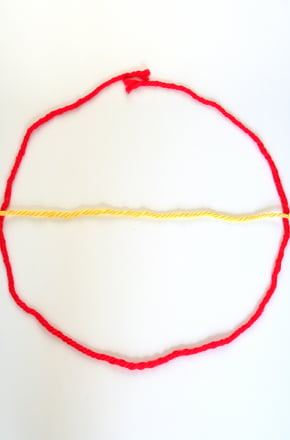# Perimeter of a Circle- Circumference

ELNA
40mins
Critical thinking, Problem Solving
Remember, Understand, Application
Yes1. Introduce the concept of circumference, radius and diameter to the students2. Introduce the concept of 'pi' and how its related with diameter and Circumference
Perimeter is the concept related to measuring the length of a straight line but when the line take a circular shape then its termed as Circumference of the circle
paper, two color strings, ruler, pen

Step1 : Suggest not to take the names of the terms that you will be introducing in class- circumference, radius and Perimeter.

Step2: Ask students to measure the piece of string with the ruler and write down the length in their note booksStep3: Help students make a triangle or square or rectangle using the piece of string, placing it on a table.

Step4: Now ask them what would be the perimeter of the shape they have created. Review the term “perimeter,” that is the length of all sides of the shape.

Step5: Ask them to make a circle with the same string. now ask them what would be the perimeter of this circle. Review the concept of perimeter again with them. Once they are clear that its the distance around the edge of the circle, then introduce the term circumference.

Step6: Explain that the length of the string is equal to the circumference, because if you were to undo the circle you would have the same length of string and the string is what comprises the distance around the circle.

Step 7: Next, review the term “diameter”. The diameter is the distance from one edge of the circle to the other that goes through the center of the circle. To show what the diameter looks like, get a second piece of string and place it in the middle of the circle from one edge to the other, cutting off any extra string that extends past the circle’s border. This straight piece of string going through the center of the circle is the diameter.

Step8: Take the diameter string off of the circle. Take a third piece of string and cut it so that it is half the length of the piece of string representing the diameter. Place this piece of string from the center of the circle to one of the edges of the circle and explain that this piece of string represents the radius. Reiterate the fact that the radius is one half the length of the diameter. You can also fold the diameter string into two equal halves and put it next to the radius string.

Step 9:Introducing the formula for getting circumference: This is where Pi needs to explained as the number which is the ratio of a circle’s circumference to its diameter. Now you can introduce the equation for circumference is 2πr.